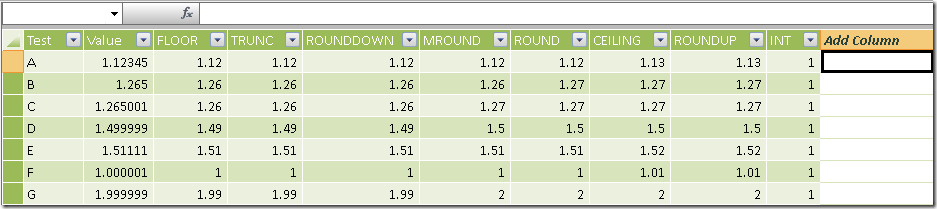Today I prepared a table of the many rounding functions available in DAX (yes, it’s part of the book we’re writing), so that I have a complete schema of the better function to use, depending on the round operation I need to do. Here is the list of functions used and then the results shown for a relevant set of values.

FLOOR = FLOOR( Tests[Value], 0.01 )

TRUNC = TRUNC( Tests[Value], 2 )

ROUNDDOWN = ROUNDDOWN( Tests[Value], 2 )

MROUND = MROUND( Tests[Value], 0.01 )

ROUND = ROUND( Tests[Value], 2 )

CEILING = CEILING( Tests[Value], 0.01 )

ROUNDUP = ROUNDUP( Tests[Value], 2 )

INT = INT( Tests[Value] )FLOOR, TRUNC and ROUNDDOWN are very similar, except on the way you can specify the number of digits to round on. On the opposite, also CEILING and ROUNDUP are very similar in their results. You can see a few differences in the way the rounding is done (see row B, where 1.265 number is rounded in two different ways on the second decimal digit) between MROUND and ROUND function.

Finally, it is important to note that FLOOR and MROUND functions don’t operate on negative numbers, while other functions do.

FLOORRounds a number down, toward zero, to the nearest multiple of significance.

FLOOR ( <Number>, <Significance> )

TRUNCTruncates a number to an integer by removing the decimal, or fractional, part of the number.

TRUNC ( <Number> [, <NumberOfDigits>] )

ROUNDDOWNRounds a number down, toward zero.

ROUNDDOWN ( <Number>, <NumberOfDigits> )

MROUNDReturns a number rounded to the desired multiple.

MROUND ( <Number>, <Multiple> )

ROUNDRounds a number to a specified number of digits.

ROUND ( <Number>, <NumberOfDigits> )

CEILINGRounds a number up, to the nearest integer or to the nearest unit of significance.

CEILING ( <Number>, <Significance> )

ROUNDUPRounds a number up, away from zero.

ROUNDUP ( <Number>, <NumberOfDigits> )

INTRounds a number down to the nearest integer.

INT ( <Number> )